\$B:#>,\$N0lGU(B

\$BKhF|!":#>,\$N0lGU\$r>e\$2\$F\$\$\$^\$9!#(B
\$B:#>,0{\$`\$b\$N\$K:\$\$C\$?\$i!";29M\$K\$7\$F\$_\$F\$/\$@\$5\$\$!#(B

2023\$BG/(B4\$B7n(B

 1(I<.0%@2Q(B 2 \$B5Y\$_(B 3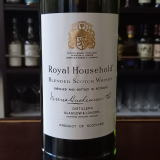(I[2TY%J3=N0YD^(B 4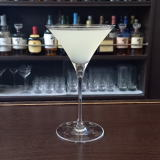(IO21P(B 5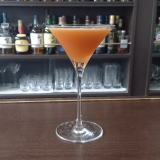(I2L^F]8^%8^[3(B 6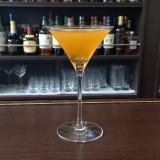(IR2C^]=^%32/<-(B 7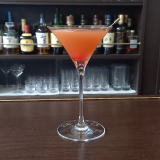(IWL^%N_0<.](B 8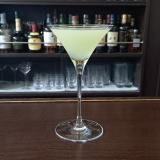(I=L_X]8^%L(0X]8^(B 9\$B5Y\$_(B 10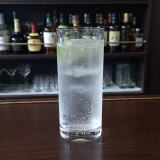(I35/6%DF/8(B 11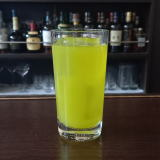(IR[]%N^0Y(B 12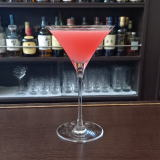(IL_XOD^]E(B 13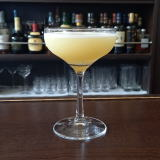(IWL^%?]8^(B 14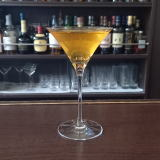(IJ^[](B 15 \$B5Y\$_(B 16 \$B5Y\$_(B 17(IK_0=LY%\0YD^(B 18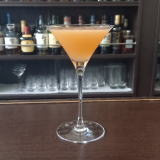(IPW8Y%K_0A(B 19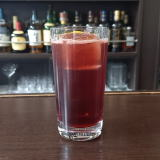(I6<=%?0@^(B 20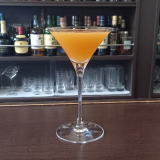(I;0D^%Z0Y(B 21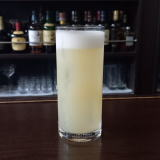(IWS=%<^]%L(=^(B 22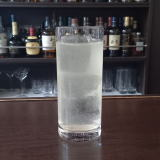(I51<=%80W0(B 23 \$B5Y\$_(B 24(IL^W]C^0%L(/8=(B 25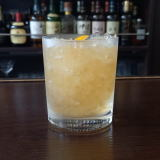(I32=70%P=D(B 26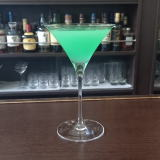(IP=D(B 27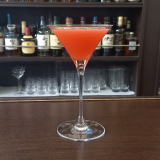(IL_[N_0=^(B 28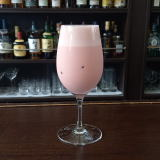(I=D[M^X0%PY8(B 29(I50YD^%L'/<.]D^(B 30 \$B5Y\$_(B